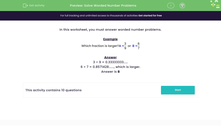# Solve A Variety of Number Problems

In this worksheet, students will solve a variety of number problems with or without the aid of a calculator.Key stage:  KS 3

Curriculum topic:   Number

Curriculum subtopic:   Use Four Operations for All Numbers

Popular topics:   Numbers worksheets

Difficulty level:#### Worksheet Overview

In this activity, we will be solving a variety of number problems.

Example

Which fraction is larger? A =
 3 9
or B =
 6 7

There are two ways we could work this out.

Method 1: Turn them into decimals.

3 ÷ 9 = 0.33333333.....

6 ÷ 7 = 0.8571428......, which is larger.

Method 2: Give the two fractions a common denominator.

The lowest common multiple of 9 and 7 is 63, so that will be our new denominator.

We multiplied the 9 by 7 to get 63, so we multiply the 3 by 7 to get 21.

We multiplied the 7 by 9 to get 63, so we multiply the 6 by 9 to get 54.

We now have A = 21/63 and B = 54/63

So, we can see that B is indeed bigger.

There is often more than one way to solve a problem - choose the one that works best for you!### What is EdPlace?

We're your National Curriculum aligned online education content provider helping each child succeed in English, maths and science from year 1 to GCSE. With an EdPlace account you’ll be able to track and measure progress, helping each child achieve their best. We build confidence and attainment by personalising each child’s learning at a level that suits them.

Get started